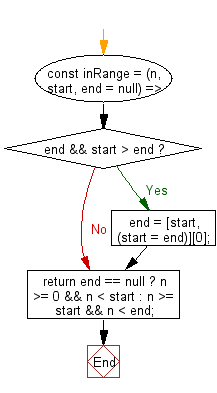# JavaScript: Check if the given number falls within the given range

## JavaScript fundamental (ES6 Syntax): Exercise-217 with Solution

Write a JavaScript program to check if the given number falls within the given range.

• Use arithmetic comparison to check if the given number is in the specified range.
• If the second argument, end, is not specified, the range is considered to be from 0 to start.

Sample Solution:

JavaScript Code:

``````//#Source https://bit.ly/2neWfJ2
const inRange = (n, start, end = null) => {
if (end && start > end) end = [start, (start = end)];
return end == null ? n >= 0 && n < start : n >= start && n < end;
};
console.log(inRange(3, 2, 5));
console.log(inRange(3, 4));
console.log(inRange(2, 3, 5));
console.log(inRange(3, 2));
```
```

Sample Output:

```true
true
false
false
```

Flowchart:Live Demo:

See the Pen javascript-basic-exercise-217-1 by w3resource (@w3resource) on CodePen.

Improve this sample solution and post your code through Disqus

What is the difficulty level of this exercise?

Test your Programming skills with w3resource's quiz.

﻿